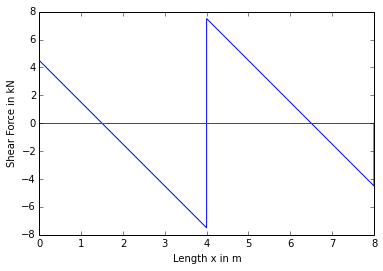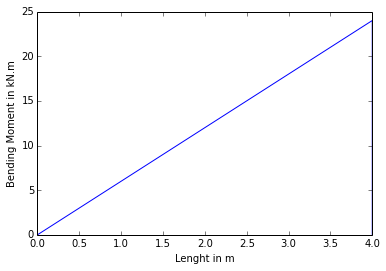# Chapter 12:Propped Cantilever¶

## Problem no 12.1,Page No.286¶

In :
import math

#Initilization of variables

L=6 #m #Length of Beam
L_1=4 #m #Length of Beam with udl Load
w=10 #KN/m #u.d.l

#Calculation

#Deflection of cantileverat C due to udl on AB
y_c=w*L_1**4*8**-1+w*L_1**3*6**-1*(L-L_1)

#Deflection of cantileverat C due to prop reaction alone
#y_c_2=R_c*L**3*3**-1

#Since both Deflection are Equal
#y_c=y_c_2

R_c=y_c*(6**3)**-1*3 #Reaction at C

#Result
print"The Reaction at End C is",round(R_c,3),"KN"

The Reaction at End C is 7.407 KN


## Problem no 12.2,Page No.286¶

In :
import math

#Initilization of variables

L=10 #m #Length
b=15 #cm #Width
d=40 #cm #Depth
y_c=1.5*10**-2 #m #Deflection
E=120*10**9
y=0.2
sigma=10*10**6 #Bending stress

#Calculations

I=b*d**3*12**-1*10**-8 #cm  #M.I

#From Deflection at the centre of cantilever we get
w=y_c*192*E*I*(L**4)**-1*10**-3 #udl distributed over the cantilever

#From Bending Moment Equation we get
w_2=sigma*I*y**-1*16*(L**2)**-1*10**-3    #udl distributed over the cantilever

#Result
print"The safe uniformly Distributed Load is",round(w_2,2),"KN/m"

The safe uniformly Distributed Load is 6.4 KN/m


## Problem no 12.3,Page No.287¶

In :
import math

#Initilization of variables

L=6 #m #span of beam
w=30*10**3 #KN/m #u.d.l

#Calculations

#Consider a section at a distance x from the fixed end A and B.M at x
#M_x=R_b*(6-x)-30*2**-1*(6-x)**2-160*(3-x)

#E*I*d**2y*(dx**2)**-1=-M_x=-R_b*(6-x)+15*(6-x)+160*(3-x)

#Now Integrating above term we get
#E*I*dy*(dx)**-1=R_b*2**-1*(6-x)**2-5*(6-x)**3-80*(3-x)**2+C_1  (Equation 1)

#Now on Integrating we get
#E*I*y=-R_b*6**-1*(6-x)**3+5*4**-1*(6-x)**2+80*3**-1*(3-x)**3+C_1*x+C_2  (Equation 2)

#At x=0,dy*dx**-1=0
#substituting in equation 1 we get
#C_1=1800-R_b

#At x=0,y=0
#substituting in equation 2 we get
#C_2=36*R_b-2340

#At x=6,y=0
R_b=72**-1*(10800-2340)

#At x=0
x=0
M_x=R_b*(6-x)-30*2**-1*(6-x)**2-160*(3-x)

#Result
print"Bending Moment at A is",round(M_x,2),"KNm"
print"The Reaction at B",round(R_b,2),"KN"

Bending Moment at A is -315.0 KNm
The Reaction at B 117.5 KN


## Problem no 12.4,Page No.288¶

In :
import math

#Initilization of variables

L=4 #span of beam
w_1=20*10**3 #Nm #u.d.l
w_2=30*10**3 #Nm #u.d.l

#Calculations

#consider a section at a distance x from A and B.M at this section is
#M_x=R_b*(3-x)-10*x**2+90*x-195

#Now integrating above equation we get
#E*I*dy*(dx)**-1=-R_b(3*x-x**2*2**-1)+10*x**3*3**-1-45*x**2+195*x+C_1

#again on Integrating we get
#E*I*y=-R_b*(3*x**2*2**-1-x**3*6**-1)+10*x**4*12**-1-15*x**3+195*x**2*2**-1+C_1*x+C_2

#At x=0,dy*(dx)**-1=0  Therefore C_1=0

#At x=0,y=0  Therefore C_2=0

#At x=3m, y=0
x=3
C_1=0
C_2=0
R_b=-(-10*x**4*12**-1+15*x**3-195*x**2*2**-1-C_1*x-C_2)*(3*x**2*2**-1-x**3*6**-1)**-1

#result

Load taken by prop is 60.0 KN


## Problem no 12.5,Page No.289¶

In :
import math

#Initilization of variables

L=2 #m #Span of beam
w=10 #KN/m #u.d.l

#Calculations

#Downward deflection at B(of Beam AB) due to u.d.l of 10 KN/m is
Y_B_1=w*L**4*8**-1

#Upward deflection at B due to reaction at C alone is
#Y_B_2=R_c*8*3**-1

#Net downward deflection of cantilever at AB at B
#Y_B=Y_B_1-Y_B_2

#Downward Deflection of Beam CD at C due to the reaction
#R_c=R_c*(3*E*I)**-1

#since both deflection at C and B are equal
R_c=20*(1*3**-1+8*3**-1)**-1

#Result
print"Reaction at C is",round(R_c,2),"KN"

Reaction at C is 6.67 KN


## Problem no 12.8,Page No.292¶

In :
import math
%matplotlib  inline

#Initilization of variables

L=8 #m #span
W=24*10**3 #N/m #U.D.L
y=2*10**-2 #m #deflection
E=20*10**9
I=10**-5 #m**4

#Calculations

#The Downward deflection at C Due to u.d.l
#Y_c=5*W*L**3*(384*E*I)**-1

#The Upward Deflection at C due to prop Reaction P
#Y_c_1=P*L**3*(48*E*I)**-1

#Since the prop is at the same level as end supports
#Y_c_1=Y_c
P_1=5*W*8**-1*10**-3 #KN

#The reaction at A and B is equal
R_a=R_b=(24-15)*2**-1

#Shear Force at B
V_B=4.5 #KN

#Shear Force at C
V_C1=4.5-24*2**-1
V_C2=4.5-24*2**-1+15

#Shea rForce at A
V_A=-4.5 #KN

#B.M at C due to u.d.l
M_C1=W*L*8**-1*10**-3 #KN*m

#B.M due to only prop reaction P=15 KN
P=15
M_C2=-P*L*4**-1 #KN*m

#B.M at D
M_D=4.5*1.5-24*8**-1*1.5**2*2**-1

#In second case prop sinks by 2 cm
#Y_c-Y_c_1=2

#So Further simplifying and sunstituting values in above equation we get
P=-(2*100**-1-(5*W*L**3*(384*E*I)**-1))*(L**3*(48*E*I)**-1)**-1

#Let Each end reaction be X
X=(24-14.625)*2**-1

#Result
print"prop reaction is",round(P_1,2),"KN"
print"The End Reaction is",round(X,2),"KN"

#Plotting the SHear Force Diagram

X1=[0,4,4,8,8]
Y1=[V_B,V_C1,V_C2,V_A,0]
Z1=[0,0,0,0,0]
plt.plot(X1,Y1,X1,Z1)
plt.xlabel("Length x in m")
plt.ylabel("Shear Force in kN")
plt.show()

#Plotting the Bendimg Moment Diagram

X2=[0,4,4]
Y2=[0,M_C1,0]
Z2=[0,0,0]
plt.plot(X2,Y2)
plt.xlabel("Lenght in m")
plt.ylabel("Bending Moment in kN.m")
plt.show()

prop reaction is 15.0 KN
The End Reaction is 4.69 KN